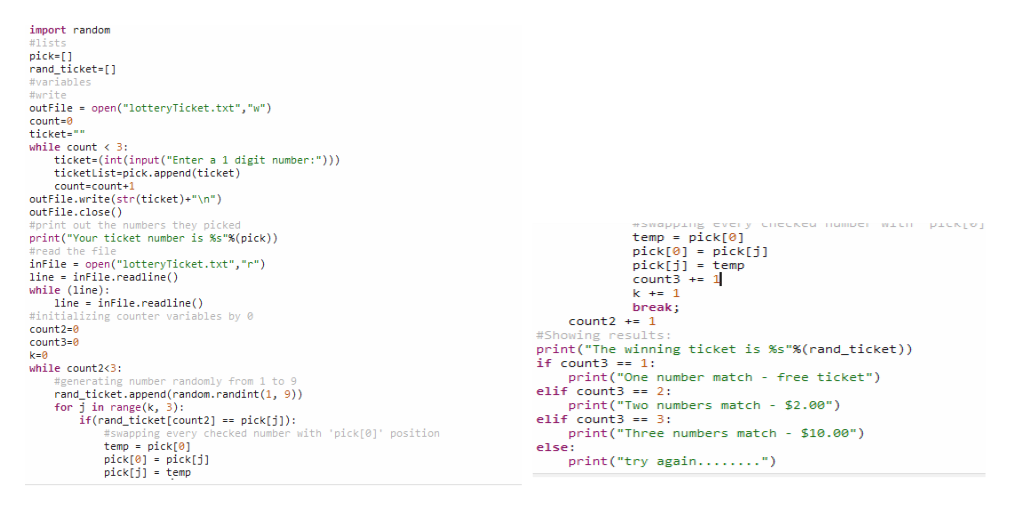# Lottery Ticket Challange- Python CodingI have coded...import random#listspick=[]rand_ticket=[]#variables#writeoutFile = open("lotteryTicket.txt","w")count=0ticket=""while count < 3:ticket=(int(input("Enter a 1 digit number:")))ticketList=pick.append(ticket)count=count+1outFile.write(str(ticket)+"\n")outFile.close()#print out the numbers they pickedprint("Your ticket number is %s"%(pick))#read the fileinFile = open("lotteryTicket.txt","r")line = inFile.readline()while (line):line = inFile.readline()count2=0while count2<3:rand_ticket.append(random.randint(1, 9))count2+=1print("The winning ticket is %s"%(rand_ticket))#compare numberscomplete this code to...Compare the text ticket and the random numbers.  If they match number (see below), output the prizeThe numbers can be in any orderthe random numbers can be duplicates, so be careful how you countOne number match - free ticketTwo numbers match  - \$2.00Three numbers match - \$10.00

Question
149 views

Lottery Ticket Challange- Python Coding

I have coded...

import random
#lists
pick=[]
rand_ticket=[]
#variables
#write
outFile = open("lotteryTicket.txt","w")
count=0
ticket=""
while count < 3:
ticket=(int(input("Enter a 1 digit number:")))
ticketList=pick.append(ticket)
count=count+1
outFile.write(str(ticket)+"\n")
outFile.close()
#print out the numbers they picked
inFile = open("lotteryTicket.txt","r")
while (line):
count2=0
while count2<3:
rand_ticket.append(random.randint(1, 9))
count2+=1
print("The winning ticket is %s"%(rand_ticket))
#compare numbers

complete this code to...

• Compare the text ticket and the random numbers.  If they match number (see below), output the prize
• The numbers can be in any order
• the random numbers can be duplicates, so be careful how you count
One number match - free ticket
Two numbers match  - \$2.00
Three numbers match - \$10.00
check_circle

Step 1

Programming Instructions:

• Create a python program and declare the required counter variables ‘count3’ and ‘k’ as 0(zero).
• Then checks for the same number in the ‘pick’ list from the generated random numbers ‘rand_ticket’ list and if any same number found then swap t...help_outlineImage Transcriptioncloseimport random #lists pick=[] rand_ticket=[) #variables #write out File = open("lotteryTicket.txt","w") count-0 ticket="" while count < 3: ticket=(int(input ("Enter a 1 digit number:"))) ticketlist=pick.append (ticket) count=count+1 out File.write(str(ticket)+"\n") out File.close() #print out the numbers they picked print("Your ticket number is %s"%(pick)) #read the file infile = open("lotteryTicket.txt","r") line = infile.readline() while (line): line = inFile.readline() #initializing counter variables by e count2=0 *SwappiLIIg CveI y LICL cu numIUCI temp - pick pick = pick[j] pick[j] = temp count3 +- 1 k += 1 %3D break; count2 += 1 #Showing results: count3=0 k=0 print ("The winning ticket is %s"%(rand_ticket)) if count3 == 1: while count2<3: print("One number match - free ticket") #generating number randomly from 1 to 9 rand_ticket.append (random.randint(1, 9)) elif count3 == 2: - \$2.00") print("Two numbers match for j in range (k, 3): if(rand_ticket[count2] == pick[j): elif count3 == 3: #swapping every checked number with 'pick' position print("Three numbers match - \$18.00") temp = pick[e] pick = pick[j] = temp else: print ("try again........") pick[j] fullscreen

### Want to see the full answer?

See Solution

#### Want to see this answer and more?

Solutions are written by subject experts who are available 24/7. Questions are typically answered within 1 hour.*

See Solution
*Response times may vary by subject and question.
Tagged in
EngineeringComputer Science

### Python